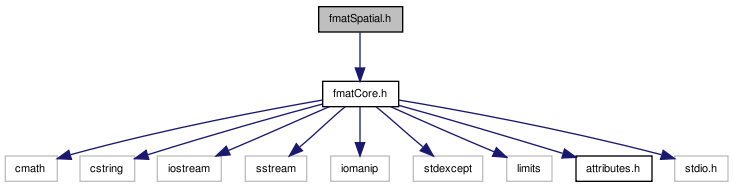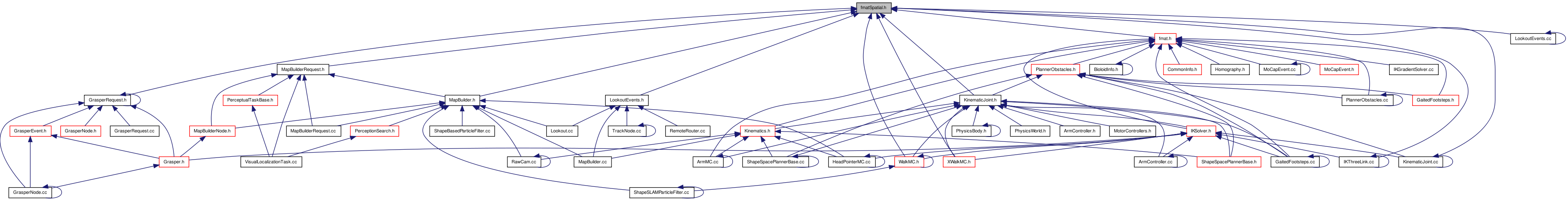# fmatSpatial.h File Reference

Provides data structures and algorithms for spatial operations. More...

`#include "fmatCore.h"`
Include dependency graph for fmatSpatial.h:This graph shows which files directly or indirectly include this file:Go to the source code of this file.

## Classes

class  fmat::QuaternionT< R >
Quaternions can be more efficient and more stable for a series of rotations than a corresponding 3x3 matrix, also more compact storage. More...
struct  fmat::fmat_internal::transformOps< R1, R2 >
Expanded calculations to facilitate optimized compilation of these operations for TransformT. More...
class  fmat::TransformT< R >
Efficient computation of affine transform operations. More...

## Namespaces

namespace  fmat

fixed-size matrix routines for high performance with small allocations

namespace  fmat::fmat_internal

## Typedefs

typedef QuaternionT fmat::Quaternion
typedef TransformT fmat::Transform

## Functions

template<typename R >
Matrix< 2, 2, R > fmat::rotation2DT (R rad)
Matrix< 2, 2, fmatRealfmat::rotation2D (fmatReal rad)
template<size_t N, typename R >
Matrix< N, N, R > fmat::rotationXN (R rad)
Matrix< 3, 3, fmatRealfmat::rotationX (fmatReal rad)
template<size_t N, typename R >
Matrix< N, N, R > fmat::rotationYN (R rad)
Matrix< 3, 3, fmatRealfmat::rotationY (fmatReal rad)
template<size_t N, typename R >
Matrix< N, N, R > fmat::rotationZN (R rad)
Matrix< 3, 3, fmatRealfmat::rotationZ (fmatReal rad)
template<class Ra , class Rb >
QuaternionT< typename
fmat_internal::promotion_trait
< Ra, Rb >::type >
fmat::crossProduct (const QuaternionT< Ra > &p, const QuaternionT< Rb > &q)
returns the rotation needed to transform p into q (this is the core of a 'slerp' implementation)
template<typename R >
QuaternionT< R > fmat::invert (const QuaternionT< R > &q)
template<typename R >
Matrix< 4, 4, R > fmat::invertTransform (const Matrix< 4, 4, R > &t)
invert the matrix, taking advantage of known structure:
template<typename R >
TransformT< R > fmat::invert (const TransformT< R > &m)
template<typename R >
Column< 3, R > fmat::ypr (const QuaternionT< R > &q)
Returns yaw-pitch-roll aka heading-elevation-bank conversion, where roll-pitch-yaw correspond to compounding rotations about the global x, y, and z axis respectively (in that order).
template<typename R >
Column< 3, R > fmat::ypr (const TransformT< R > &m)
Returns yaw-pitch-roll aka heading-elevation-bank conversion, where roll-pitch-yaw correspond to compounding rotations about the global x, y, and z axis respectively (in that order).
template<template< size_t, size_t, typename R > class M, typename R >
Column< 3, typename
fmat_internal::unconst< R >
::type >
fmat::ypr (const M< 3, 3, R > &m)
Returns yaw-pitch-roll aka heading-elevation-bank conversion, where roll-pitch-yaw correspond to compounding rotations about the global x, y, and z axis respectively (in that order).
template<typename R >
Column< 2, R > fmat::segmentIntersection (const Column< 2, R > &p1, const Column< 2, R > &d1, const Column< 2, R > &p2, const Column< 2, R > &d2)
Returns the scaling factors for d1 and d2 through p1 and p2 respectively to reach common intersection.
template<typename R >
fmat::rayIntersection (const Column< 2, R > &p1, const Column< 2, R > &d1, const Column< 2, R > &p2, const Column< 2, R > &d2)
Returns the scaling factor of d1 from p1 to reach intersection of d2 through p2.
template<typename R >
Column< 2, R > fmat::lineIntersection (const Column< 2, R > &p1, const Column< 2, R > &d1, const Column< 2, R > &p2, const Column< 2, R > &d2)
Returns the point of intersection of d1 through p1 and d2 through p2.
template<template< size_t, typename R > class V, typename R >
Column< 2, typename V< 2, R >
::out_t >
fmat::normalLeft (const V< 2, R > &v)
Returns the orthogonal left vector (rotate by 90°).
template<template< size_t, typename R > class V, typename R >
Column< 2, typename V< 2, R >
::out_t >
fmat::normalRight (const V< 2, R > &v)
Returns the orthogonal right vector (rotate by -90°).

## Detailed Description

Provides data structures and algorithms for spatial operations.

Definition in file fmatSpatial.h.

 Tekkotsu v5.1CVS Generated Mon May 9 04:58:56 2016 by Doxygen 1.6.3